## Mathematical Puzzles

In order to develop our logical thinking, it's essential to exercise our brain from childhood. Brain teasers and logical puzzles would be of much help here. These websites offer numerous mathematical riddles that are going to challenge your brain! Furthermore, you can find here puzzles of different levels to suit adults and kids.

## Mathematical Puzzles selection: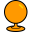Sporcle - A World of Quizzes

Play millions of free online trivia quizzes. There is a fun quiz about virtually every topic imaginable: Geography, History, Sports, Music, TV and more!
Math is Fun

Math explained in easy language, plus puzzles, games, worksheets and an illustrated dictionary. For K-12 kids, teachers and parents.Math Worksheets | K-8 Math Resources for Teachers

Math Worksheets | K-8 Math Resources for Teachers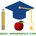Basic Mathematics

Do not spend lots of money on courses and software! My website is designed to give you a solid understanding of basic mathematics, algebra, and geometry.Lateral Thinking and Logic Puzzles

Logic puzzles and lateral thinking puzzles organized by difficulty and type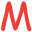Thousands of free math worksheets, math IQ puzzles and math educational resources for math students ...

Thousands of math worskheets and brain teasers for primary and elementary math education based on the Singapore math curriculum
Kids Math Games Online - Free Interactive Learning Activities, Fun Educational Resources

Enjoy an awesome range of free math games, interactive activities, practice exercises and cool problem solving challenges that are perfect for kids learning math as well as teachers looking for fun, educational resources online.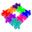Fun With Puzzles

Solve many types of puzzles like number puzzles, fun puzzles, maths brain teasers, crack-the-code puzzles, logic puzzles, visual puzzles, and riddles.
Genius Puzzles and Riddles

GPuzzles is directed towards the people interested in puzzles, riddles, and quiz and is suitable for a wide array of people as they cater for all IQ levels.
Math and Science For Children - Pre - K , Kindergarten to 7th Grades | Math 4 Children Plus

Math and science for kids- Resources: math worksheets, flash cards, math games For 1st, 2nd, 3rd, 4th, 5th, 6th, 7th grades - Homeschool Parent and Teachers.

## Additional websites, related to Mathematical Puzzles:

In domain
In search queries
Filters
Relevant Popular
Nick's Mathematical Miscellany

Miscellaneous resources and links, including Nick's Mathematical Puzzles.
Cryptarithms - Number Puzzles - Cryptarithms.com

Cryptarithms are mathematical puzzles in which the digits in an arithmetic expression are replaced by letters of the alphabet. The object is to work out which numbers correspond to each letter. Generally the letters are chosen so that the sum also sp...
Forward Junction Puzzles

Mathematics Puzzles and Malayalam IQ Questions
Puzzles | Barryispuzzled

Logic and mathematics puzzles from polymath Barry R. Clarke otherwise known as barryispuzzled
Magic Square Puzzles | Stop fearing Mathematics and Start loving Mathematics

Magic Square Puzzles are Arithmetic and Logic puzzles. Your children would soon do all the addition, subtraction in their mind itself. Welcome to Magic Square Puzzles, the one and only Puzzle based on the Magic squares. Magic square Puzzles are bein...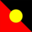Mathematics Centre: ... A world of alternatives to text-based learning

Mathematics Centre brings together Mathematics Task Centre, Calculating Changes, Picture Puzzles, Maths300 and more in a world of alternatives to help students at all levels learn to work like a mathematician. Managed by Black Douglas Professional Ed...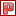Logicville : Puzzles and Brainteasers

Logicville is a website collection of mathematical games, puzzles, and brain teasers, including anagrams, cryptograms, cryptarithms or alphametics, word puzzles, logic games, doublets, tangrams, sudoku, chess problems, and many educational and math p...